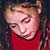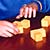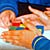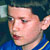Magic Mathworks Travelling CircusExperiencing Mathematics through Sight, Sound, Touch and Movement# Coxeter’s Cake: solution

## Coxeter’s Cake: solution

The square is a ‘tangential’ polygon: it has an incircle.

The area of a tangential polygon is rs, where r is the inradius; s, half the perimeter.

The area of any slice cut from the incentre is therefore proportional to the length of perimeter between the cuts.

So all you have to do is measure the perimeter and divide it into 5 equal parts.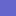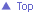The Magic Mathworks Travelling Circus